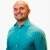# SAT Prep How to determine if a point lies on a lineThis lesson will look at an example question on the SAT involving lines. The given information will assist in determining if a point lies on a line. This lesson will give a step by step explanation... This lesson will look at an example question on the SAT involving lines. The given information will assist in determining if a point lies on a line. This lesson will give a step by step explanation to better explain the concept.
More... Collapse
9 Views